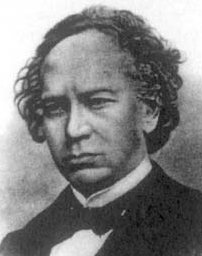A# Hermite, Charles (1822–1901)Charles Hermite was a French mathematician whose work in the theory of functions includes the application of elliptic functions to provide the first solution to the general equation of the fifth degree, the quintic equation. He also showed that e is a transcendental number, studied a class of differential equations now known as Hermite polynomials, which later proved to be of importance in some applications of quantum mechanics, and discovered the properties of Hermitian matrices.